Next: 1.2 Device Efficiency Up: 1. Introduction Previous: 1. Introduction   Contents

# 1.1 Fundamentals of Thermoelectrics

Thermoelectric phenomena in materials can be described through three thermoelectric effects, the Seebeck effect, the Peltier effect, and the Thomson effect. The Seebeck effect, discovered in 1821 by Thomas Johann Seebeck , represents the generation of an electromotive force by a temperature gradient. When a temperature gradient is applied along a conductive material, charge carriers move from the hot to the cold side. In the case of open-circuit, charge accumulation results in an electric potential difference, as shown in Fig. 1.2. The Seebeck coefficient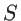of a material shows the magnitude of the induced voltage in response to the temperature difference: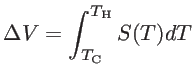(1.1)

where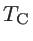and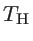are the temperatures of the cold and the hot side, respectively. The Seebeck coefficient of a material is generally a function of temperature. However, if the temperature difference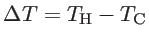is small enough, and the Seebeck coefficient is nearly constant in the range of applied temperatures, one has: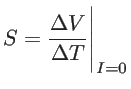(1.2)

A single thermoelectric conductor, however, is not able to play the role of a battery. This is due to the fact that the net loop voltage would be zero if circuit wires of the same conductor were connected. A non-zero loop voltage can be obtained when two dissimilar conductors are connected in the configuration shown in Fig. 1.3. The circuit configuration of a thermoelectric generator is shown in Fig. 1.4-a. Here the generator is composed of two materials, an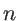-type and a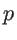-type material. The voltage obtained from this configuration is: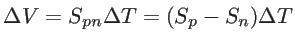(1.3)

where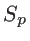and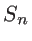are the Seebeck coefficients of the-type and-type materials, respectively, and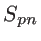is the Seebeck coefficient of the device (junction). The Seebeck coefficient of a-type material is positive, that of an-type material negative. Therefore, using this configuration one can achieve a high net voltage.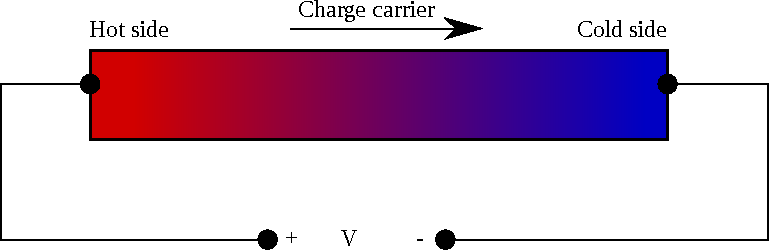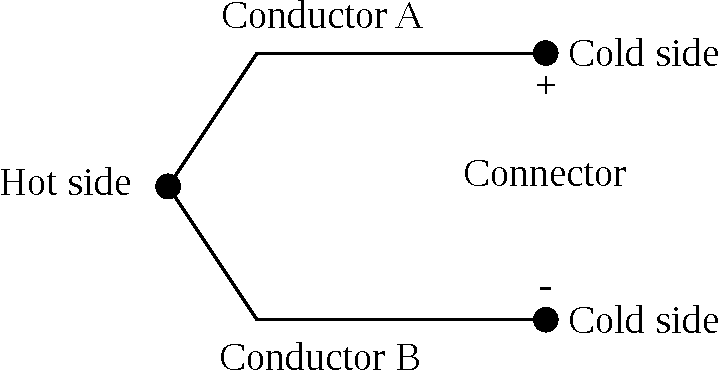The Peltier effect, on the other hand, discovered by Athanaseal Jean Charles Peltier in 1834, describes how an electrical current can create a heat flow. This effect has enabled the second application of thermoelectric devices, the thermoelectric cooler, as shown in Fig. 1.4-b. Here, by applying an external power source, both the electrons of the-type conductor and the holes of the-type conductor move and carry heat from one side to the other. Therefore, one side cools down, whereas the other side heats up. The heat flow absorbed at the hot side is proportional to the current through the junction: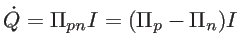(1.4)

where,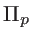,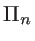, and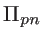are the Peltier coefficients of the-type material, the-type material, and the device, respectively .

The Thomson effect, observed by William Thomson in 1851, expresses a relation for heat production in a current-carrying conductor in the presence of temperature gradient: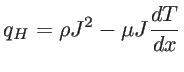(1.5)

where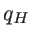is the heat production per volume,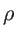the electrical resistivity,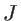the current density, and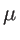the Thomson coefficient. Considering the second term in Eq. 1.5, the conductor can either release or absorb the heat, depending on the direction of current with respect to the temperature gradient.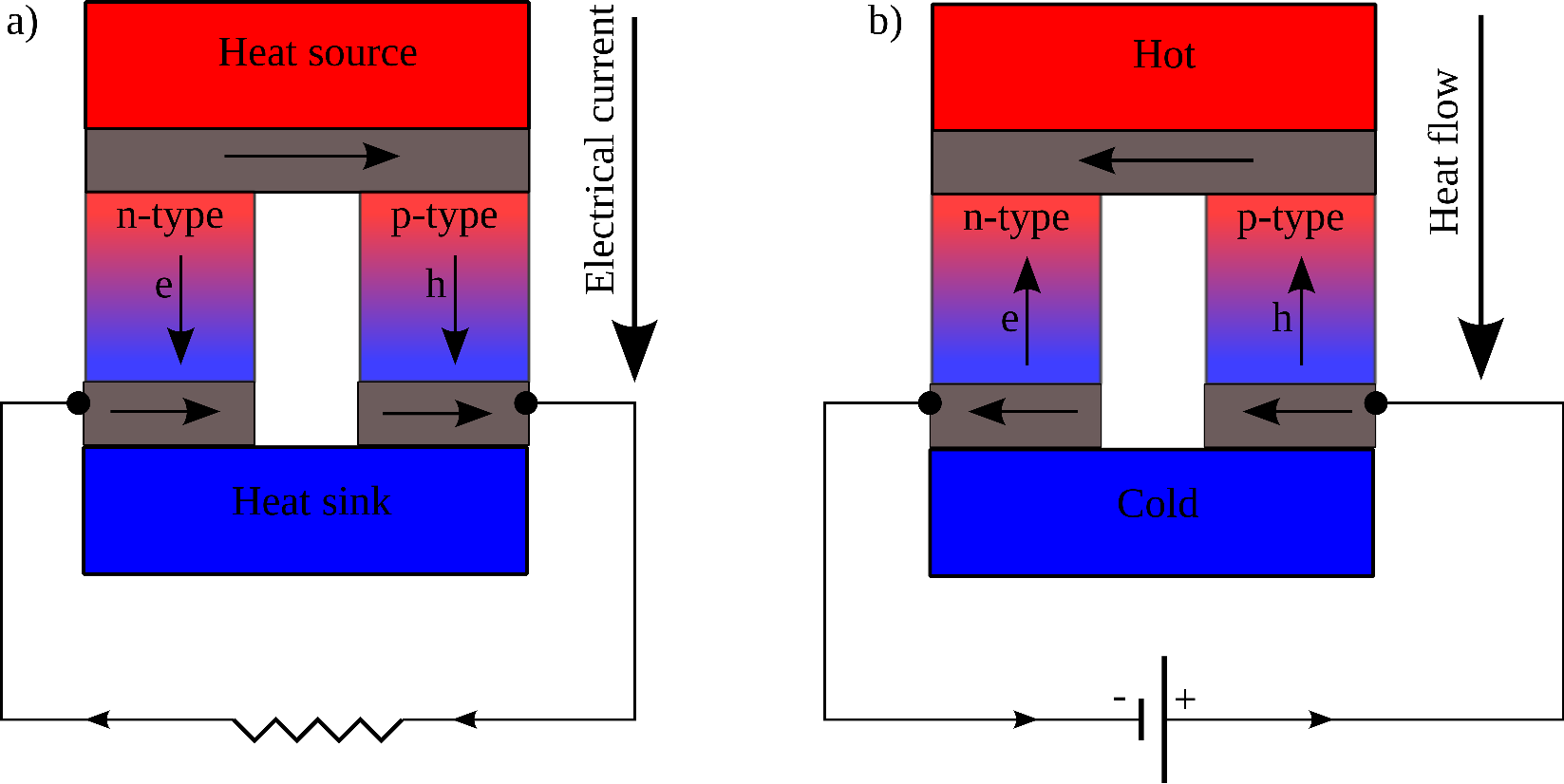According to the Thomson relations, these three thermoelectric coefficients are not independent. The Peltier coefficient and the Seebeck coefficient are linearly related to each other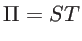(1.6)

whereas the Thomson coefficient can be expressed as :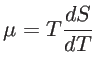(1.7)

Therefore, the Seebeck coefficient as a function of temperature is enough for describing all three thermoelectric phenomena. In Chapter 2, the computational method for obtaining the Seebeck coefficient, as well as the calculation of the other transport coefficients, is presented. It is, however, worth mentioning that one can only measure the Seebeck coefficient for a pair (junction) of dissimilar materials, but not of an individual conductor, whereas measurement of the Thomson coefficient is possible even for individual materials.Next: 1.2 Device Efficiency Up: 1. Introduction Previous: 1. Introduction   Contents
H. Karamitaheri: Thermal and Thermoelectric Properties of Nanostructures